# Constants

These Constants blocks are special Number blocks that hold mathematical constants when the blocks are created.

Block Icon Description
Pi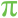A Number block that holds the mathematical constant pi
E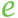A Number block that holds the mathematical constant e
SQRT2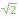A Number block that holds the mathematical constant for the square root of 2
LN2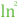A Number block that holds the mathematical constant for the natural logarithm of 2
LN10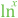A Number block that holds the mathematical constant for the natural logarithm of 10
LOG2E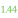A Number block that holds the mathematical constant for the base-2 logarithm of the constant e
LOG10E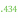A Number block that holds the mathematical constant for the base-10 logarithm of the constant e

2018/10/03 00:17